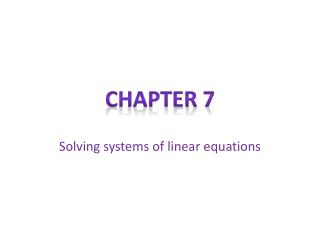DownloadDownload PresentationSolving systems of linear equations

# Solving systems of linear equations

Download Presentation## Solving systems of linear equations

- - - - - - - - - - - - - - - - - - - - - - - - - - - E N D - - - - - - - - - - - - - - - - - - - - - - - - - - -
##### Presentation Transcript

1. Chapter 7 Solving systems of linear equations

2. Entry Task 02/17/2011 • Get 7.1B and a piece of graph paper • Get out 6.4 so I can check it for group points. • Copy the below information onto a sheet of paper.

3. Section 7.1 • Objective: Solve systems of linear equations by graphing. Model a real life problem using a linear system.

4. Entry Task 02/12/2013Write the following vocabulary in your math notebook • A system of linear equations in two variables is two linear equations with two variables. • A solution of a system of linear equations in two variables is an ordered pair (x,y) that satisfies each equation in the system. • If the two lines do not cross (parallel) then there is no solution. • If the two lines do cross there is one solution. • If the two lines are the same the answer is all real numbers {(x,y); }

5. Et 02/05/2013 copy this down: Solving systems of two linear Equations by graphing • 1.) Solve each equation for y • 2.) Graph each equation (use a ruler!) • 3.) Find the point of intersection • This is your solution 4.) Check your answer • If the two lines do not cross (parallel) then there is no solution. • If the two lines do cross there is one solution. • If the two lines are the same the answer is all real numbers {(x,y); }

6. Solving a system of linear equations by elimination • Step 1: make sure all of your variables are lined up • Step 2: make sure a variable will cancel out if you add the equations; if not multiply • Step 3: add the two equations • Step 4: Solve for the remaining variable • Step 5: find the other variable • Step 6: write your answer in the form (x,y)

7. Example • Solve the linear system. {

8. Section 7.2 • Objective: Use substitution to solve a linear system. Model a real life problem using a linear system.

9. Solving a linear system by substitution • Step 1: solve one of the equations for one of its variables. • Step 2: substitute the expression from step one into the other equation and solve for the other variable. • Step 3: Substitute the value from step 2 into the revised equation from step one and solve. • Step 4: check the solution in each of the original equations.

10. Example • Solve the linear system. { • Step 1: solve for y in equation 1. • Step 2: Substitute for y in equation 2 and solve for x.

11. Example cont. • Step 3: to find the value of y, substitute -1 for x in the revised Equation 1. • Step 4: check that (-1,0) is a solution by substituting -1 for x and 0 for y in each of the original equations. • The solution is (-1,0)

12. Entry Task 02/24/2011 • Get out 7.2B so I can check it for group points. • Solve the following system of equations using substitution, if you need help look on page 405 in your book. {

13. Entry Task 02/25/2011 • Get out 7.2B so I can check it for group points. • Complete 7.2 Standardized Test Practice problems 1-7. You have 14 minutes. • When finished get a book and worksheet 7.3B and take notes for section 7.3 on the back of the worksheet. 7.3B is due on Tuesday.

14. Entry Task 02/28/2011 • Get out 7.3B and prepare any questions you may have. • If you have no questions start working on problems 1-12, and 19-25 • 13-18 will be extra credit.

15. Entry Task 03/01/2011 • Get out 7.3B to check for group points • Do numbers 1 and 2 on 7.3 stp • Get a book and open to page 360, start taking notes.

16. Entry Task 03/02/2011 • Get out pg. 363 #1-6 to check for group points • Get a book and on your homework paper, do problem number 7 on pg. 363.

17. Section 7.6 • Objective: Solve a system of linear inequalities by graphing. Use a system of linear inequalities to model a real-life problem. • Open your books to page 432

18. Entry Task 03/03/2011 • Get out pg. 435 # 1-8 to check for group points • Work on your homework and prepare any questions you may have. If you are finished start on pg. 435 #9-17, 34-35.

19. Entry Task 03/04/2011 • Choose one of the following: • Work on missing work • Take the Quiz. • If you are not working you will have to take the quiz.